• numpy 矩阵乘法NumPy matrix multiplication can be done by the following three methods. NumPy矩阵乘法可以通过以下三种方法完成。 multiply(): element-wise matrix multiplication. multiple（）：逐元素矩阵...

numpy矩阵乘法

NumPy matrix multiplication can be done by the following three methods.
NumPy矩阵乘法可以通过以下三种方法完成。
multiply(): element-wise matrix multiplication. multiple（）：逐元素矩阵乘法。 matmul(): matrix product of two arrays. matmul（）：两个数组的矩阵乘积。 dot(): dot product of two arrays. dot（）：两个数组的点积。
1. NumPy矩阵乘法元素明智 (1. NumPy Matrix Multiplication Element Wise)
If you want element-wise matrix multiplication, you can use multiply() function.
如果要逐元素矩阵相乘，可以使用multiple（）函数。

import numpy as np

arr1 = np.array([[1, 2],
[3, 4]])
arr2 = np.array([[5, 6],
[7, 8]])

arr_result = np.multiply(arr1, arr2)

print(arr_result)
Output:
输出：
[[ 5 12]
[21 32]]
The below image shows the multiplication operation performed to get the result matrix.
下图显示了为获得结果矩阵而执行的乘法运算。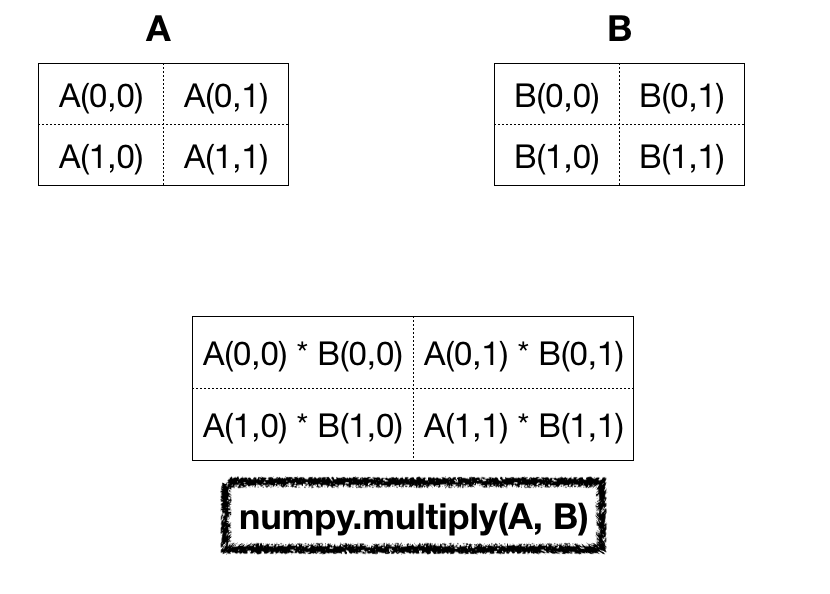Numpy Matrix multiply()
numpy矩阵乘法（）

2.两个NumPy阵列的矩阵乘积 (2. Matrix Product of Two NumPy Arrays)
If you want the matrix product of two arrays, use matmul() function.
如果要两个数组的矩阵乘积，请使用matmul（）函数。

import numpy as np

arr1 = np.array([[1, 2],
[3, 4]])
arr2 = np.array([[5, 6],
[7, 8]])

arr_result = np.matmul(arr1, arr2)

print(f'Matrix Product of arr1 and arr2 is:\n{arr_result}')

arr_result = np.matmul(arr2, arr1)

print(f'Matrix Product of arr2 and arr1 is:\n{arr_result}')
Output:
输出：

Matrix Product of arr1 and arr2 is:
[[19 22]
[43 50]]
Matrix Product of arr2 and arr1 is:
[[23 34]
[31 46]]
The below diagram explains the matrix product operations for every index in the result array. For simplicity, take the row from the first array and the column from the second array for each index. Then multiply the corresponding elements and then add them to reach the matrix product value.
下图说明了结果数组中每个索引的矩阵乘积运算。 为简单起见，为每个索引取第一个数组的行和第二个数组的列。 然后乘以相应的元素，然后将它们相加以达到矩阵乘积值。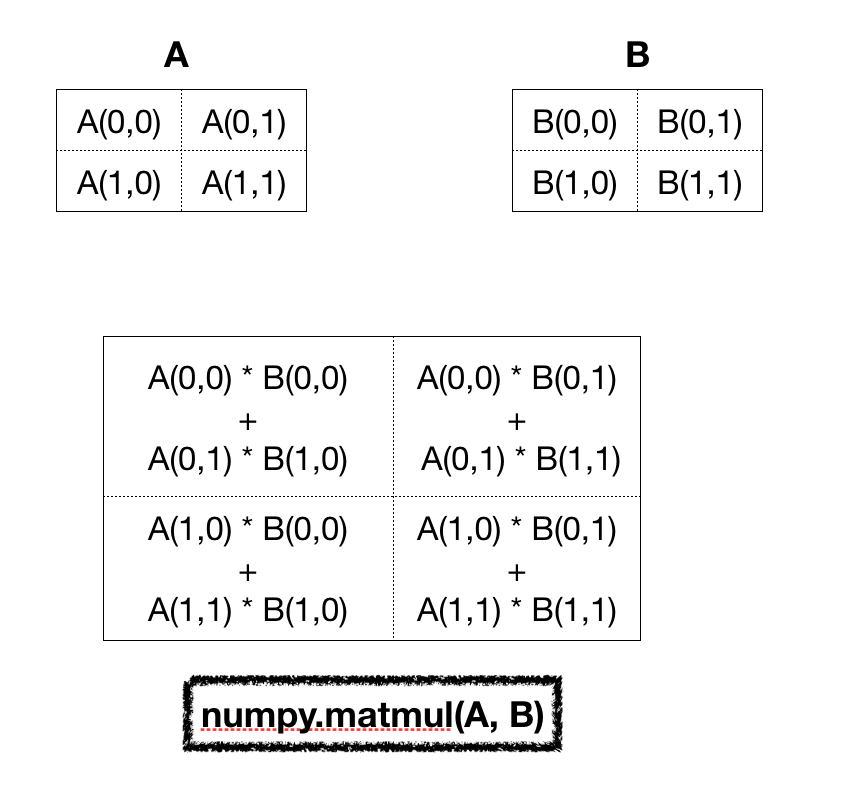Numpy Matrix Product
块状矩阵产品

The matrix product of two arrays depends on the argument position. So matmul(A, B) might be different from matmul(B, A).
两个数组的矩阵乘积取决于参数位置。 因此，matmul（A，B）可能与matmul（B，A）不同。
3.两个NumPy阵列的点积 (3. Dot Product of Two NumPy Arrays)
The numpy dot() function returns the dot product of two arrays. The result is the same as the matmul() function for one-dimensional and two-dimensional arrays.
numpy dot（）函数返回两个数组的点积。 结果与一维和二维数组的matmul（）函数相同。
import numpy as np

arr1 = np.array([[1, 2],
[3, 4]])
arr2 = np.array([[5, 6],
[7, 8]])

arr_result = np.dot(arr1, arr2)

print(f'Dot Product of arr1 and arr2 is:\n{arr_result}')

arr_result = np.dot(arr2, arr1)

print(f'Dot Product of arr2 and arr1 is:\n{arr_result}')

arr_result = np.dot([1, 2], [5, 6])
print(f'Dot Product of two 1-D arrays is:\n{arr_result}')
Output:
输出：
Dot Product of arr1 and arr2 is:
[[19 22]
[43 50]]
Dot Product of arr2 and arr1 is:
[[23 34]
[31 46]]
Dot Product of two 1-D arrays is:
17

numpy.square() numpy.square（） NumPy sqrt() – Square Root of Matrix Elements NumPy sqrt（）–矩阵元素的平方根 Python NumPy Tutorial Python NumPy教程

参考资料 ()
展开全文python 机器学习 matlab php
• [Numpy]array与matrix乘法上的不同1 Numpy乘法的实现2 上代码（array，matrix在*，dot，multiply上的异同）2.1 定义array和matrix两个矩阵2.2 取array和matrix的第一行2.3 取array和matrix的第一列2.4 array进行...


[Numpy]array与matrix在乘法上的不同
1 Numpy乘法的实现2 上代码（array，matrix在*，dot，multiply上的异同）2.1 定义array和matrix两个矩阵2.2 取array和matrix的第一行2.3 取array和matrix的第一列2.4 array进行乘法运算（*，dot，multiply）2.5 matrix进行乘法运算（*，dot，multiply）

1 Numpy乘法的实现
运算解释*array:对应元素相乘，matrix:矩阵相乘np.dot矩阵相乘np.multiply对应元素相乘
2 上代码（array，matrix在*，dot，multiply上的异同）
2.1 定义array和matrix两个矩阵
import numpy as np

array = np.array([[1,2],[3,4]])
matrix = np.matrix('1 2;3 4')
print('array')
print(array)
print('matrix')
print(matrix)
print('-' * 20)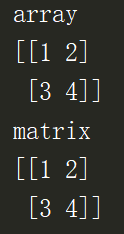2.2 取array和matrix的第一行
print('array:')
print(array)
print('matrix:')
print(matrix)
print('-' * 20)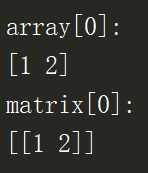注：matrix只要取到的最终结果不是数字，就会保留二维矩阵的形式，但array不会，array是一个行向量
2.3 取array和matrix的第一列
print('array[:,0]')
print(array[:,0])
print('matrix[:,0]')
print(matrix[:,0])
print('-' * 20)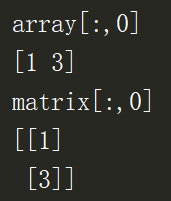array[:,0]–>返回的是一个行向量，对应array的第0列的列向量，而matrix[;,0]以矩阵的形式保存列向量
2.4 array进行乘法运算（*，dot，multiply）
print(array)
print(array * array)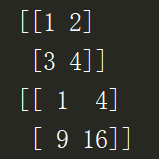在array中*为对应元素相乘

a

r

r

a

y

=

[

1

2

3

4

]

array = \begin{bmatrix} 1 & 2 \\ 3 & 4 \end{bmatrix}

a

r

r

a

y

∗

a

r

r

a

y

=

[

1

⋅

1

2

⋅

2

3

⋅

3

4

⋅

4

]

=

[

1

4

9

16

]

array * array = \begin{bmatrix} 1\cdot1 & 2\cdot2 \\ 3\cdot3 & 4\cdot4 \end{bmatrix} = \begin{bmatrix} 1 & 4 \\ 9 & 16 \end{bmatrix}

print(np.multiply(array,array))

注：此处np.multiply(array,array) 等价于 array * array
print(array * array)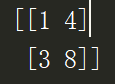a

r

r

a

y

=

[

1

2

3

4

]

array = \begin{bmatrix} 1 & 2 \\ 3 & 4 \\ \end{bmatrix}

a

r

r

a

y

[

0

]

=

[

1

2

]

array = \begin{bmatrix} 1 & 2 \end{bmatrix}

a

r

r

a

y

∗

a

r

r

a

y

[

0

]

=

n

p

.

m

u

l

t

i

p

l

y

(

a

r

r

a

y

,

a

r

r

a

y

[

0

]

)

=

[

1

⋅

1

2

⋅

2

3

⋅

1

4

⋅

2

]

=

[

1

4

3

8

]

array * array = np.multiply(array,array) = \begin{bmatrix} 1\cdot1 & 2\cdot2 \\ 3\cdot1 & 4\cdot2 \end{bmatrix}=\begin{bmatrix} 1 & 4 \\ 3 & 8 \end{bmatrix}

print(array * np.array([array[:,0]]).T)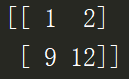a

r

r

a

y

=

[

1

2

3

4

]

array = \begin{bmatrix} 1 & 2 \\ 3 & 4 \\ \end{bmatrix}

n

p

.

a

r

r

a

y

(

[

a

r

r

a

y

[

:

,

0

]

]

)

.

T

=

[

1

3

]

np.array([array[:,0]]).T = \begin{bmatrix} 1\\ 3\\ \end{bmatrix}

a

r

r

a

y

∗

n

p

.

a

r

r

a

y

(

[

a

r

r

a

y

[

:

,

0

]

]

)

.

T

=

[

1

⋅

1

2

⋅

1

3

⋅

3

4

⋅

3

]

=

[

1

2

9

12

]

array * np.array([array[:,0]]).T = \begin{bmatrix} 1\cdot1 & 2\cdot1 \\ 3\cdot3 & 4\cdot3 \\ \end{bmatrix} = \begin{bmatrix} 1 & 2 \\ 9 & 12 \end{bmatrix}

print(np.dot(array,array))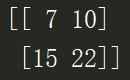a

r

r

a

y

=

[

1

2

3

4

]

array = \begin{bmatrix} 1 & 2 \\ 3 & 4 \\ \end{bmatrix}

n

p

.

d

o

t

(

a

r

r

a

y

,

a

r

r

a

y

)

=

[

1

⋅

1

+

2

⋅

3

1

⋅

2

+

2

⋅

4

3

⋅

1

+

4

⋅

3

3

⋅

2

+

4

⋅

4

]

=

[

7

10

15

22

]

np.dot(array,array) = \begin{bmatrix} 1\cdot 1+2\cdot3 & 1\cdot2 + 2\cdot4\\ 3\cdot1 + 4\cdot3 & 3\cdot2 + 4\cdot4 \end{bmatrix} = \begin{bmatrix} 7 & 10 \\ 15 & 22 \end{bmatrix}

2.5 matrix进行乘法运算（*，dot，multiply）
print(matrix * matrix)
print(np.dot(matrix,matrix))


m

a

t

r

i

x

=

[

1

2

3

4

]

matrix = \begin{bmatrix} 1 & 2 \\ 3 & 4 \\ \end{bmatrix}

n

p

.

d

o

t

(

m

a

t

r

i

x

,

m

a

t

r

i

x

)

=

m

a

t

r

i

x

∗

m

a

t

r

i

x

=

[

1

⋅

1

+

2

⋅

3

1

⋅

2

+

2

⋅

4

3

⋅

1

+

4

⋅

3

3

⋅

2

+

4

⋅

4

]

=

[

7

10

15

22

]

np.dot(matrix ,matrix ) = matrix * matrix = \begin{bmatrix} 1\cdot 1+2\cdot3 & 1\cdot2 + 2\cdot4\\ 3\cdot1 + 4\cdot3 & 3\cdot2 + 4\cdot4 \end{bmatrix} = \begin{bmatrix} 7 & 10 \\ 15 & 22 \end{bmatrix}

print(
np.multiply(matrix  matrix)
)


m

a

t

r

i

x

=

[

1

2

3

4

]

matrix = \begin{bmatrix} 1 & 2 \\ 3 & 4 \\ \end{bmatrix}

n

p

.

m

u

l

t

i

p

l

y

(

m

a

t

r

i

x

,

m

a

t

r

i

x

)

=

[

1

⋅

1

2

⋅

2

3

⋅

3

4

⋅

4

]

=

[

1

4

9

16

]

np.multiply(matrix ,matrix )= \begin{bmatrix} 1\cdot1 & 2\cdot2 \\ 3\cdot3 & 4\cdot4 \end{bmatrix} = \begin{bmatrix} 1 & 4 \\ 9 & 16 \end{bmatrix}


展开全文multiply 矩阵相乘
• ## NumPy 矩阵乘法

万次阅读 多人点赞 2018-05-23 17:23:37
NumPy 支持的几类矩阵乘法也很重要。 元素级乘法 你已看过了一些元素级乘法。你可以使用 multiply 函数或 * 运算符来实现。回顾一下，它看起来是这样的： m = np.array([[1,2,3],[4,5,6]]) m # 显示以下结果： ...
NumPy 支持的几类矩阵乘法也很重要。
元素级乘法
你已看过了一些元素级乘法。你可以使用 multiply 函数或 * 运算符来实现。回顾一下，它看起来是这样的：
m = np.array([[1,2,3],[4,5,6]])
m
# 显示以下结果：
# array([[1, 2, 3],
#        [4, 5, 6]])

n = m * 0.25
n
# 显示以下结果：
# array([[ 0.25,  0.5 ,  0.75],
#        [ 1.  ,  1.25,  1.5 ]])

m * n
# 显示以下结果：
# array([[ 0.25,  1.  ,  2.25],
#        [ 4.  ,  6.25,  9.  ]])

np.multiply(m, n)   # 相当于 m * n
# 显示以下结果：
# array([[ 0.25,  1.  ,  2.25],
#        [ 4.  ,  6.25,  9.  ]])
矩阵乘积
要获得矩阵乘积，你可以使用 NumPy 的 matmul 函数。
如果你有兼容的形状，那就像这样简单：
a = np.array([[1,2,3,4],[5,6,7,8]])
a
# 显示以下结果：
# array([[1, 2, 3, 4],
#        [5, 6, 7, 8]])
a.shape
# 显示以下结果：
# (2, 4)

b = np.array([[1,2,3],[4,5,6],[7,8,9],[10,11,12]])
b
# 显示以下结果：
# array([[ 1,  2,  3],
#        [ 4,  5,  6],
#        [ 7,  8,  9],
#        [10, 11, 12]])
b.shape
# 显示以下结果：
# (4, 3)

c = np.matmul(a, b)
c
# 显示以下结果：
# array([[ 70,  80,  90],
#        [158, 184, 210]])
c.shape
# 显示以下结果：
# (2, 3)
如果你的矩阵具有不兼容的形状，则会出现以下错误：
np.matmul(b, a)
# 显示以下错误：
# ValueError: shapes (4,3) and (2,4) not aligned: 3 (dim 1) != 2 (dim 0)
NumPy 的 dot 函数
有时候，在你以为要用 matmul 函数的地方，你可能会看到 NumPy 的 dot 函数。事实证明，如果矩阵是二维的，那么 dot 和 matmul 函数的结果是相同的。
所以这两个结果是等价的：
a = np.array([[1,2],[3,4]])
a
# 显示以下结果：
# array([[1, 2],
#        [3, 4]])

np.dot(a,a)
# 显示以下结果：
# array([[ 7, 10],
#        [15, 22]])

a.dot(a)  # you can call你可以直接对 ndarray 调用 dot
# 显示以下结果：
# array([[ 7, 10],
#        [15, 22]])

np.matmul(a,a)
# array([[ 7, 10],
#        [15, 22]])
虽然这两个函数对于二维数据返回相同的结果，但在用于其他数据形状时，你应该谨慎选择。你可以在 matmul和 dot 文档中详细了解它们的差异，并找到其他 NumPy 函数的链接。
展开全文数据处理
• matrix和array的乘法有所区别 1、array A * A是对应元素相乘，np.dot(A, A)是矩阵乘法 2、matrix ...A * A是矩阵乘法，np.multiply(A, A)是对应元素相乘 ...具体参考这篇博文[numpy]matrix和array的乘和加...
matrix和array的乘法有所区别
1、array
A * A是对应元素相乘，np.dot(A, A)是矩阵乘法
2、matrix
A * A是矩阵乘法，np.multiply(A, A)是对应元素相乘
具体参考这篇博文[numpy]matrix和array的乘和加
展开全文python array
• 讨论numpy中的np.matmul，.dot以及*的区别，要区分一维情况和二维情况。 一维情况 import numpy as np np_array = np.arange(3) print( 'np_array:', np_array, '\ndot:', np_array.dot(np_array), '\n*:', np_...机器学习
• Numpy中矩阵乘法问题对于array对象对于matrix对象总结 Numpy中的矩阵乘法分为两大情况，使用numpy.array和使用numpy.matrix. Numpy确实重载了*操作符，可以直接对array或者matrix对象进行乘法运算，但是在不同对象...
• 两者相似但执行相同的运算可能得到不同的结果 显然，array只能通过dot()实现“矩阵乘法”，...而matrix则不同，可以直接使用"*"运算符实现“矩阵乘法”，如下图： 注意，我们在数据处理中使用较多的是array。 ...array
• 结论： 元素乘法：np.multiply(a,b) ...唯独注意：*，在 np.array 中重载为元素乘法，在 np.matrix 中重载为矩阵乘法! 对于 np.array 对象 >>> a array([[1, 2], [3, 4]]) array中对应元素乘法。...
• 由于需要求数组的逆，而numpy.ndarray不能直接求逆，需要先转换为numpy.matrix类型。 ndarray转matrix： x = np.mat(x) matrix转ndarray: x = x.A 需要注意的是，matrix 只能表示二维数据，用其他维度的ndarray...
• numpy 向量乘法Prerequisite: Defining Vector using Numpy 先决条件： 使用Numpy定义Vector To read about the Scalar Multiplication of Vector, visit: Scalar Multiplication of Vector 要了解向量的标量乘法...python 机器学习 线性代数 深度学习
• ## Python Numpy矩阵乘法

千次阅读 2020-09-15 18:41:23
In this tutorial we will see python matrix multiplication using numpy (Numerical Python) library. 在本教程中，我们将看到使用numpy(Numerical Python)库的python矩阵乘法 。 For using numpy you must ...数据结构 python 算法 机器学习
• 文章主要介绍了 Numpy 中几个乘法函数的使用方法，包含较多的代码示例，建议点击目录直达所需内容。在以下示例代码中，均已事前导入Numpy：import numpy as np 版本 Python 3.7.0 Numpy 1.18.1 np.multiply(x1, x2...python 函数
• matrix()和array()关于秩的区别 运算的区别 两个对象之间的相互转化 生成方式的区别 初始化特定值的矩阵，我们一般用到的方式是np.mat()或np.array()。相信使用过numpy库的学者都应该接触过这两种方法...
• NumPy的主要对象是同种元素的多维数组。这是一个所有的元素都是一种类型、通过一个正整数元组索引的元素...在用python求线代中的秩中，我们用numpy包中的linalg.matrix_rank方法计算矩阵的秩。 import num...Python
• np.array、np.matrix两种类对象可以进行矩阵乘法运算，但是同样的 “*”在两种对象里的含义不一样。要么全部使用np.array，要么全部使用np.matrix，别弄混淆了。。。
• ## numpymatrix 矩阵对象

千次阅读 2017-04-26 16:52:04
https://docs.scipy.org/doc/numpy/reference/generated/numpy.matrix.html#numpy.matrix 1.简介 Matrix类型继承于ndarray类型，因此含有ndarray的所有数据属性和方法。Matrix类型与ndarray类型有六个重要的不同点...对象 matlab 继承
• Numpy matrices必须是2维的,但是numpy arrays (ndarrays) 可以是多维的（1D，2D，3D···...在numpymatrix的主要优势是：相对简单的乘法运算符号。例如，a和b是两个matrices，那么a*b，就是矩阵积。 import num...arrays
• 6.numpy 中的array与numpy中的matrix的最大的不同是，在做归约运算时，array的维数会发生变化，但matrix总是保持为2维。例如下面求平均值的运算 >>> C matrix( [[1, 2], [3, 4]] ) >>> C.mean( 1 ) matrix( [...
• NumPy中最重要的类ndarray和matrix，理解它们的四则运算至关重要，然而它们跟数学中的矩阵运算还是有一定差别。ndarray 点乘
• Python中的几种矩阵乘法 1. 同线性代数中矩阵乘法的定义： np.dot() np.dot(A, B)：对于二维矩阵，计算真正意义上的矩阵乘积，同线性代数中矩阵乘法的定义。...two_dim_matrix_one = np.array([[1, 2, 3]...
• *：在 np.array 中重载为元素乘法，在 np.matrix 中重载为矩阵乘法 np.matrix： 必须是2维的 numpy arrays (ndarrays)： 可以是任意多维的（1D，2D，3D····ND） 即：matrix 是 array 的一个小的分支，包含于 ...python 算法
• np.dot()即线性代数中的乘法 python代码如下： import numpy as np # 2-D array: 2 x 3 two_dim_matrix_one = np.array([[1, 2, 3], [4, 5, 6]]) # 2-D array: 3 x 2 two_dim_matrix_two = np.array([[1, 2], [3...
• matrix', 'division', 'euler_from_matrix', 'euler_from_quaternion', 'euler_matrix', 'identity_matrix', 'inverse_matrix', 'is_same_transform', 'math', 'numpy', 'orthogonalization_matrix', 'projection_...
• 文章目录前言一、 NumpyMatrix二、实战案例1.Matrix 前言 一、 NumpyMatrix 一、 NumpyMatrix 1.NumPy函数库中存在两种不同的数据类型(矩阵matrix和数组array)，都可以用于处理行 列表示的数字元素。虽然它们...python
• NumPy的主要对象是同种元素的多维数组。这是一个所有的元素都是一种类型、通过一个正整数元组...在NumPy中维度(dimensions)叫做轴(axes)，轴的个数叫做秩(rank，但是和线性代数中的秩不是一样的，在用python求python
• ## <Python> Numpy中的矩阵乘法问题

万次阅读 多人点赞 2017-07-27 22:48:54
最近参加的一个Program，主题是生物识别，其中的PCA/LDA特征值提取部分需要大量用到线性...Numpy中的矩阵乘法分为两大情况，使用numpy.array和使用numpy.matrix. Numpy确实重载了*操作符，可以直接对array或者matrixpython
• 想要实现线性代数中讲过的矩阵乘法，即符合矩阵相乘的两个矩阵需要满足条件：第一个矩阵的列数等于第二个矩阵的行数。使用numpy的库函数：np.matmul。如果参与matmul运算的两个矩阵尺寸不满足上述条件则会报错 a = ...
• 元素乘法：np.multiply(a,b) 矩阵乘法：np.dot(a,b) 或 np.matmul(a,b) 或 a.dot(b) 或直接用 a @ b ! 唯独注意：*，在 np.array 中重载为元素乘法，在 np.matrix 中重载为矩阵乘法)
• 使用numpy过程中，经常涉及mat和array的使用、相互转换，用的糊里糊涂，一不小心就报错。现在就系统的梳理下两者的用法和关系。 matrix与array的关系 matrix是np.array的子集，mat是只能是二维矩阵，当做线代的矩阵...
• 详解numpy中array类型和matrix类型 Numpy中两大重要类型array类型(N维数组ndarray)和矩阵类型matrix是很多基于Numpy数值计算的基础，因此搞清楚两者的关系是相当重要的， 特别是程序中混杂了这两种类型，还夹带着...ndarray python 转化...